<![CDATA[Forum of Mathematics, Pi]]>https://www.cambridge.org/core/journals/forum-of-mathematics-pi/latest-issueForum of Mathematics, Pi20505086Cambridge University Press<![CDATA[Brill-Noether theory for curves of a fixed gonality]]>

We prove a generalisation of the Brill-Noether theorem for the variety of special divisors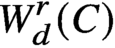on a general curve C of prescribed gonality. Our main theorem gives a closed formula for the dimension of. We build on previous work of Pflueger, who used an analysis of the tropical divisor theory of special chains of cycles to give upper bounds on the dimensions of Brill-Noether varieties on such curves. We prove his conjecture, that this upper bound is achieved for a general curve. Our methods introduce logarithmic stable maps as a systematic tool in Brill-Noether theory. A precise relation between the divisor theory on chains of cycles and the corresponding tropical maps theory is exploited to prove new regeneration theorems for linear series with negative Brill-Noether number. The strategy involves blending an analysis of obstruction theories for logarithmic stable maps with the geometry of Berkovich curves. To show the utility of these methods, we provide a short new derivation of lifting for special divisors on a chain of cycles with generic edge lengths, proved using different techniques by Cartwright, Jensen, and Payne. A crucial technical result is a new realisability theorem for tropical stable maps in obstructed geometries, generalising a well-known theorem of Speyer on genus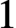curves to arbitrary genus.

]]>
https://dx.doi.org/10.1017/fmp.2020.14?rft_dat=source%3Ddrssdoi:10.1017/fmp.2020.14Brill-Noether theory for curves of a fixed gonality110.1017/fmp.2020.14https://dx.doi.org/10.1017/fmp.2020.14?rft_dat=source%3Ddrss2021-01-08Forum of Mathematics, Pi2021-01-08Jensen, DavidRanganathan, Dhruv
<![CDATA[The fields of values of characters of degree not divisible by p]]>

We study the fields of values of the irreducible characters of a finite group of degree not divisible by a prime p. In the case where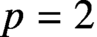, we fully characterise these fields. In order to accomplish this, we generalise the main result of [ILNT] to higher irrationalities. We do the same for odd primes, except that in this case the analogous results hold modulo a simple-to-state conjecture on the character values of quasi-simple groups.

]]>
https://dx.doi.org/10.1017/fmp.2021.1?rft_dat=source%3Ddrssdoi:10.1017/fmp.2021.1The fields of values of characters of degree not divisible by p210.1017/fmp.2021.1https://dx.doi.org/10.1017/fmp.2021.1?rft_dat=source%3Ddrss2021-02-15Forum of Mathematics, Pi2021-02-15Navarro, GabrielTiep, Pham Huu
<![CDATA[Holomorphic anomaly equation for $({\mathbb P}^2,E)$ and the Nekrasov-Shatashvili limit of local ${\mathbb P}^2$]]>

We prove a higher genus version of the genuslocal-relative correspondence of van Garrel-Graber-Ruddat: for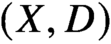a pair with X a smooth projective variety and D a nef smooth divisor, maximal contact Gromov-Witten theory ofwith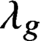-insertion is related to Gromov-Witten theory of the total space of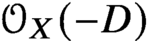and local Gromov-Witten theory of D.

Specializing tofor S a del Pezzo surface or a rational elliptic surface and E a smooth anticanonical divisor, we show that maximal contact Gromov-Witten theory of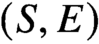is determined by the Gromov-Witten theory of the Calabi-Yau 3-foldand the stationary Gromov-Witten theory of the elliptic curve E.

Specializing further to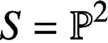, we prove that higher genus generating series of maximal contact Gromov-Witten invariants of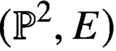are quasimodular and satisfy a holomorphic anomaly equation. The proof combines the quasimodularity results and the holomorphic anomaly equations previously known for local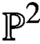and the elliptic curve.

Furthermore, using the connection between maximal contact Gromov-Witten invariants of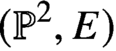and Betti numbers of moduli spaces of semistable one-dimensional sheaves on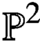, we obtain a proof of the quasimodularity and holomorphic anomaly equation predicted in the physics literature for the refined topological string free energy of localin the Nekrasov-Shatashvili limit.

]]>
https://dx.doi.org/10.1017/fmp.2021.3?rft_dat=source%3Ddrssdoi:10.1017/fmp.2021.3Holomorphic anomaly equation for $({\mathbb P}^2,E)$ and the Nekrasov-Shatashvili limit of local ${\mathbb P}^2$310.1017/fmp.2021.3https://dx.doi.org/10.1017/fmp.2021.3?rft_dat=source%3Ddrss2021-05-03Forum of Mathematics, Pi2021-05-03Bousseau, PierrickFan, HongluGuo, ShuaiWu, Longting
<![CDATA[Simultaneously vanishing higher derived limits]]>

In 1988, Sibe Mardešić and Andrei Prasolov isolated an inverse system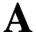with the property that the additivity of strong homology on any class of spaces which includes the closed subsets of Euclidean space would entail that(the nth derived limit of) vanishes for every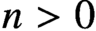. Since that time, the question of whether it is consistent with the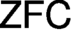axioms that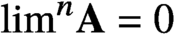for every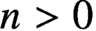has remained open. It remains possible as well that this condition in fact implies that strong homology is additive on the category of metric spaces.

We show that assuming the existence of a weakly compact cardinal, it is indeed consistent with theaxioms that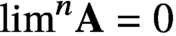for all. We show this via a finite-support iteration of Hechler forcings which is of weakly compact length. More precisely, we show that in any forcing extension by this iteration, a condition equivalent to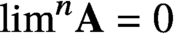will hold for each. This condition is of interest in its own right; namely, it is the triviality of every coherent n-dimensional family of certain specified sorts of partial functionswhich are indexed in turn by n-tuples of functions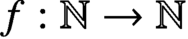. The triviality and coherence in question here generalise the classical and well-studied case of.

]]>
https://dx.doi.org/10.1017/fmp.2021.4?rft_dat=source%3Ddrssdoi:10.1017/fmp.2021.4Simultaneously vanishing higher derived limits410.1017/fmp.2021.4https://dx.doi.org/10.1017/fmp.2021.4?rft_dat=source%3Ddrss2021-06-14Forum of Mathematics, Pi2021-06-14Bergfalk, JeffreyLambie-Hanson, Chris